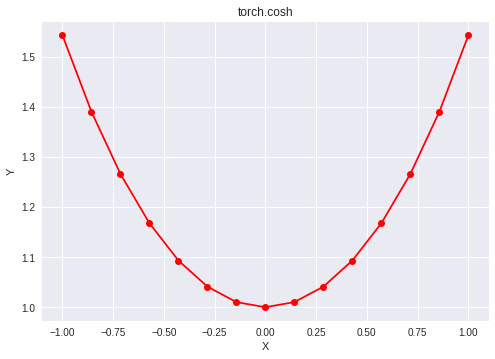# Python | PyTorch cosh() method

• Last Updated : 05 Jan, 2022

PyTorch is an open-source machine learning library developed by Facebook. It is used for deep neural network and natural language processing purposes.

The function `torch.cosh()` provides support for the hyperbolic cosine function in PyTorch. It expects the input in radian form. The input type is tensor and if the input contains more than one element, element-wise hyperbolic cosine is computed.

Syntax: torch.cosh(x, out=None)

Parameters:
x: Input tensor
name (optional): Output tensor

Return type: A tensor with the same type as that of x.

Code #1:

## Python3

 `# Importing the PyTorch library ` `import` `torch ` ` `  `# A constant tensor of size 6 ` `a ``=` `torch.FloatTensor([``1.0``, ``-``0.5``, ``3.4``, ``-``2.1``, ``0.0``, ``-``6.5``]) ` `print``(a) ` ` `  `# Applying the cosh function and ` `# storing the result in 'b' ` `b ``=` `torch.cosh(a) ` `print``(b) `

Output:

``` 1.0000
-0.5000
3.4000
-2.1000
0.0000
-6.5000
[torch.FloatTensor of size 6]

1.5431
1.1276
14.9987
4.1443
1.0000
332.5716
[torch.FloatTensor of size 6]
```

Code #2: Visualization

## Python3

 `# Importing the PyTorch library ` `import` `torch ` ` `  `# Importing the NumPy library ` `import` `numpy as np ` ` `  `# Importing the matplotlib.pyplot function ` `import` `matplotlib.pyplot as plt ` ` `  `# A vector of size 15 with values from -1 to 1 ` `a ``=` `np.linspace(``-``1``, ``1``, ``15``) ` ` `  `# Applying the hyperbolic cosine function and ` `# storing the result in 'b' ` `b ``=` `torch.cosh(torch.FloatTensor(a)) ` ` `  `print``(b) ` ` `  `# Plotting ` `plt.plot(a, b.numpy(), color ``=` `'red'``, marker ``=` `"o"``)  ` `plt.title(``"torch.cosh"``)  ` `plt.xlabel(``"X"``)  ` `plt.ylabel(``"Y"``)  ` ` `  `plt.show() `

Output:

``` 1.5431
1.3904
1.2661
1.1678
1.0933
1.0411
1.0102
1.0000
1.0102
1.0411
1.0933
1.1678
1.2661
1.3904
1.5431
[torch.FloatTensor of size 15]
```My Personal Notes arrow_drop_up
Recommended Articles
Page :